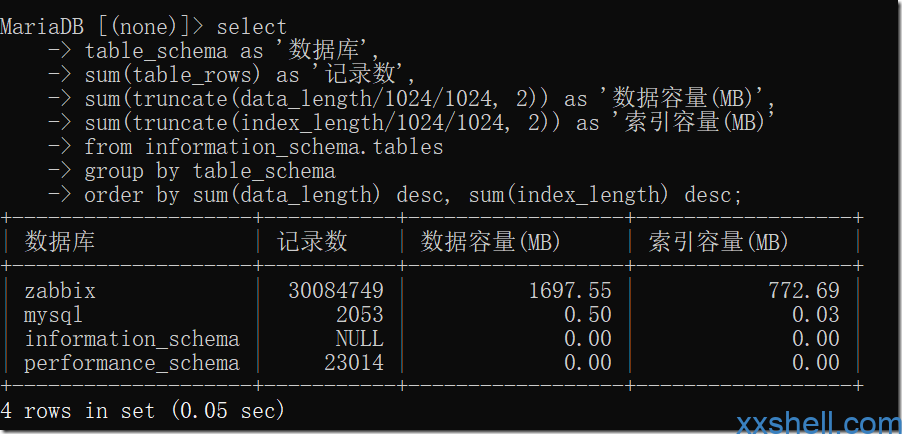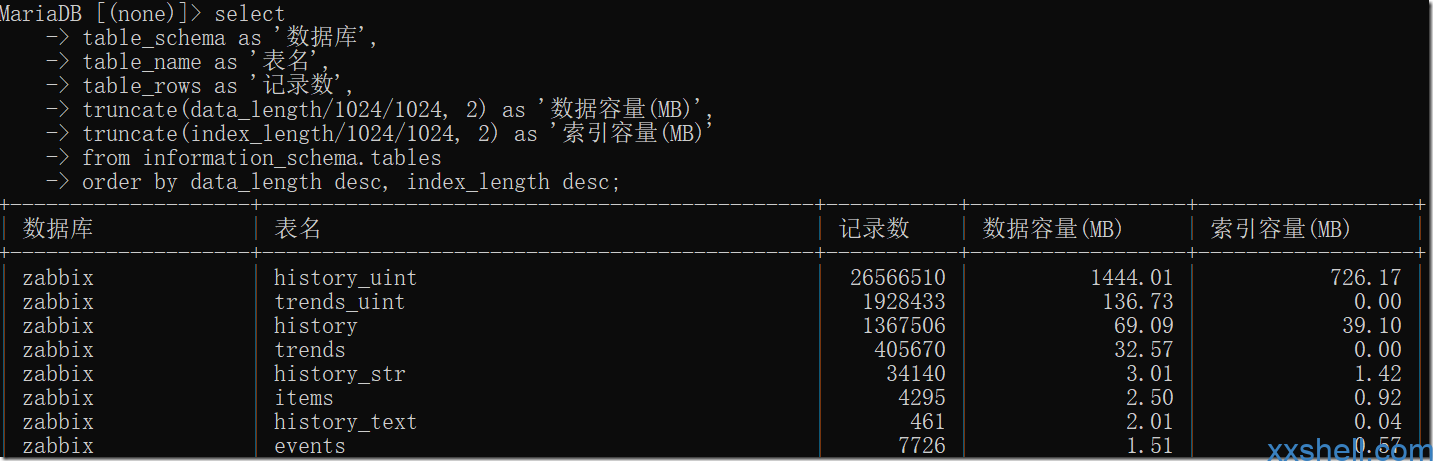## 1、查看所有数据库容量大小

```select
table_schema as '数据库',
sum(table_rows) as '记录数',
sum(truncate(data_length/1024/1024, 2)) as '数据容量(MB)',
sum(truncate(index_length/1024/1024, 2)) as '索引容量(MB)'
from information_schema.tables
group by table_schema
order by sum(data_length) desc, sum(index_length) desc;```## 2、查看所有数据库各表容量大小

```select
table_schema as '数据库',
table_name as '表名',
table_rows as '记录数',
truncate(data_length/1024/1024, 2) as '数据容量(MB)',
truncate(index_length/1024/1024, 2) as '索引容量(MB)'
from information_schema.tables
order by data_length desc, index_length desc;```## 3、查看指定数据库容量大小（zabbix为例）

```select
table_schema as '数据库',
sum(table_rows) as '记录数',
sum(truncate(data_length/1024/1024, 2)) as '数据容量(MB)',
sum(truncate(index_length/1024/1024, 2)) as '索引容量(MB)'
from information_schema.tables
where table_schema='zabbix';```## 4、查看指定数据库各表容量大小（zabbix为例）

```select
table_schema as '数据库',
table_name as '表名',
table_rows as '记录数',
truncate(data_length/1024/1024, 2) as '数据容量(MB)',
truncate(index_length/1024/1024, 2) as '索引容量(MB)'
from information_schema.tables
where table_schema='zabbix'
order by data_length desc, index_length desc;```• 回顶